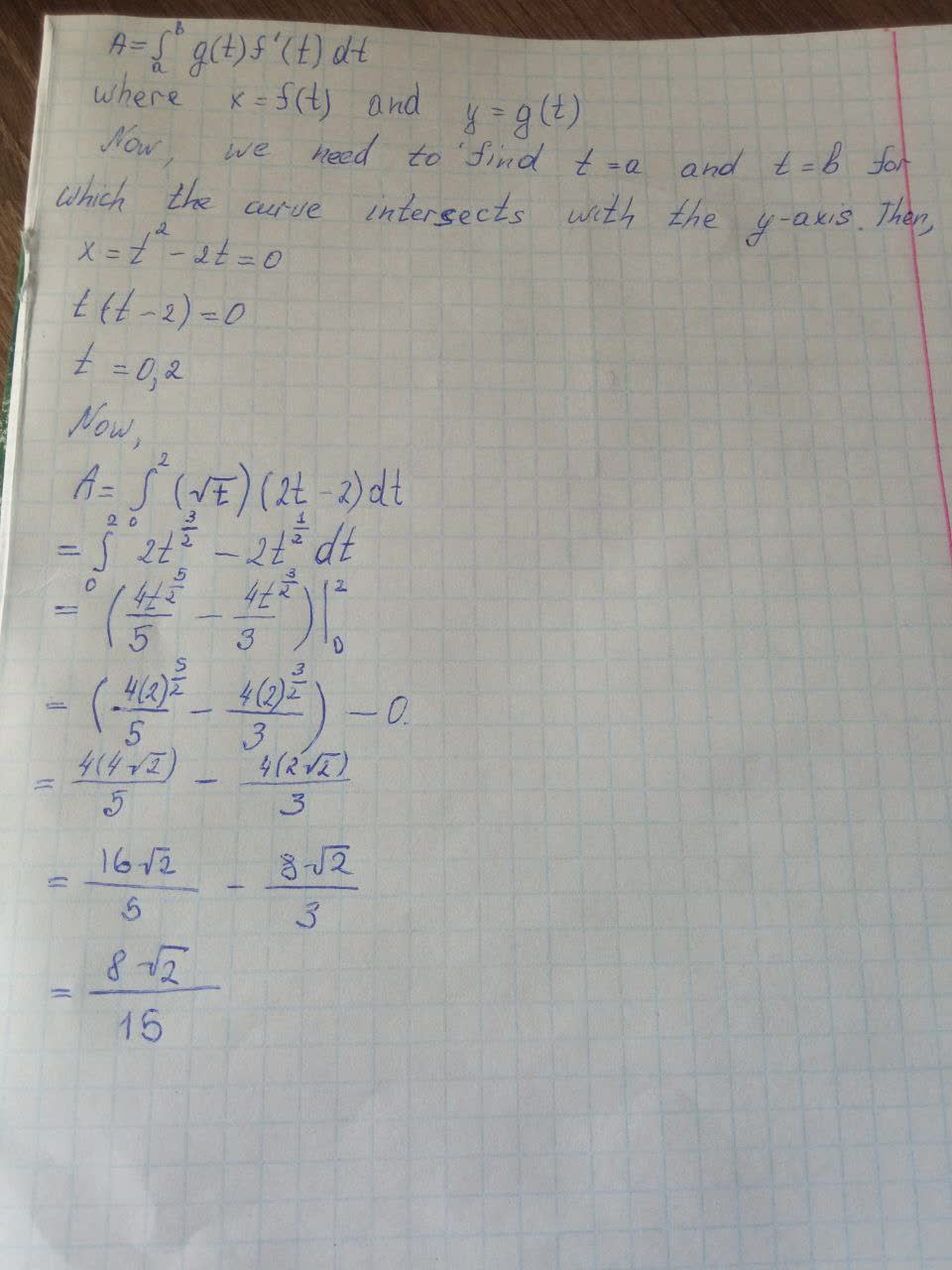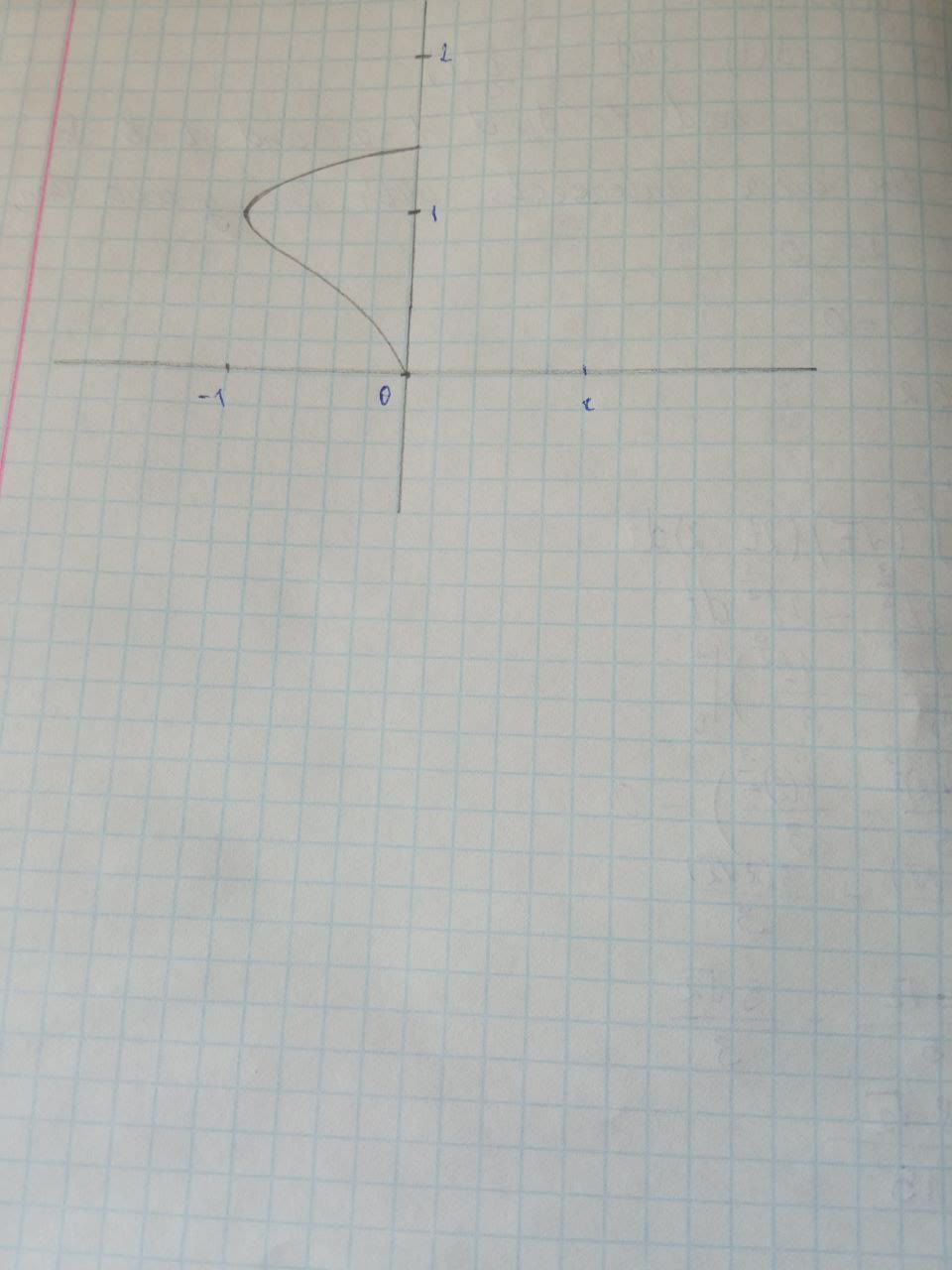Find the area enclosed by the curve x=t^2-2t,\ y=\sqrt{t} andUkusakazaL 2021-08-16 Answered
Find the area enclosed by the curve $$\displaystyle{x}={t}^{{2}}-{2}{t},\ {y}=\sqrt{{{t}}}$$ and the y-axis

• Questions are typically answered in as fast as 30 minutes

Solve your problem for the price of one coffee

• Math expert for every subject
• Pay only if we can solve itBrighton
To find the area enclosed by the curve: